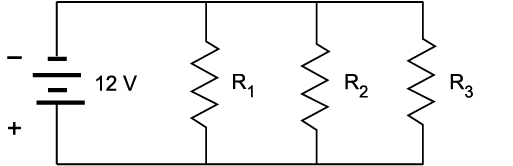# 7.2: Parallel Circuits

•• Camosun College
$$\newcommand{\vecs}{\overset { \rightharpoonup} {\mathbf{#1}} }$$ $$\newcommand{\vecd}{\overset{-\!-\!\rightharpoonup}{\vphantom{a}\smash {#1}}}$$$$\newcommand{\id}{\mathrm{id}}$$ $$\newcommand{\Span}{\mathrm{span}}$$ $$\newcommand{\kernel}{\mathrm{null}\,}$$ $$\newcommand{\range}{\mathrm{range}\,}$$ $$\newcommand{\RealPart}{\mathrm{Re}}$$ $$\newcommand{\ImaginaryPart}{\mathrm{Im}}$$ $$\newcommand{\Argument}{\mathrm{Arg}}$$ $$\newcommand{\norm}{\| #1 \|}$$ $$\newcommand{\inner}{\langle #1, #2 \rangle}$$ $$\newcommand{\Span}{\mathrm{span}}$$ $$\newcommand{\id}{\mathrm{id}}$$ $$\newcommand{\Span}{\mathrm{span}}$$ $$\newcommand{\kernel}{\mathrm{null}\,}$$ $$\newcommand{\range}{\mathrm{range}\,}$$ $$\newcommand{\RealPart}{\mathrm{Re}}$$ $$\newcommand{\ImaginaryPart}{\mathrm{Im}}$$ $$\newcommand{\Argument}{\mathrm{Arg}}$$ $$\newcommand{\norm}{\| #1 \|}$$ $$\newcommand{\inner}{\langle #1, #2 \rangle}$$ $$\newcommand{\Span}{\mathrm{span}}$$$$\newcommand{\AA}{\unicode[.8,0]{x212B}}$$

The parallel circuit is probably the most common type of circuit you will encounter. Loads in power distribution systems are usually connected in parallel with each other in one way or another. A parallel circuit is constructed by connecting the terminals of all the individual load devices so that the same value of voltage appears across each component. In Figure $$\PageIndex{1}$$, you can see that each of the three resistors receives the same voltage from the source.Figure $$\PageIndex{1}$$: A parallel circuit (CC BY-NC-SA; BC Industry Training Authority)

Figure $$\PageIndex{2}$$ shows the more traditional schematic of the same circuit. Notice that:

• The total supply voltage appears across each of the three resistors.
• There are three separate paths (or branches) for current flow, each leaving the negative terminal of the supply and returning to the positive terminal.Figure $$\PageIndex{2}$$: Schematic of a parallel circuit (CC BY-NC-SA; BC Industry Training Authority)

The two fundamental characteristics of any parallel circuit are that:

• The voltage across each branch is the same.
• There is more than one path for the current to flow through.

In contrast to a series circuit, current still flows to the remaining devices in the circuit if any one branch or component in a parallel circuit is opened.